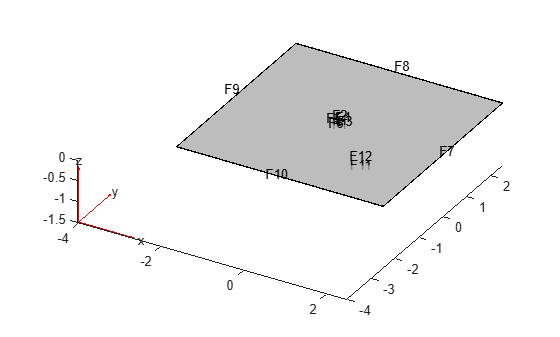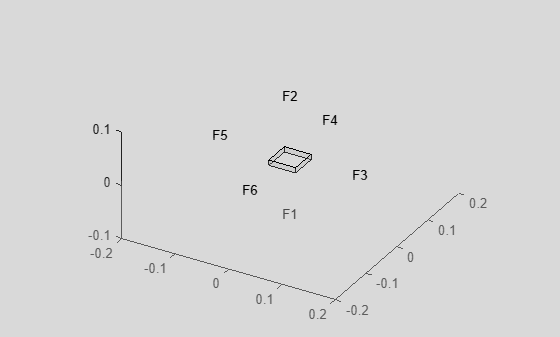Documentation

# TransientStructuralResults

Transient structural solution and its derived quantities

## Description

A `TransientStructuralResults` object contains the displacement, velocity, and acceleration in a form convenient for plotting and postprocessing.

Displacement, velocity, and acceleration are reported for the nodes of the triangular or tetrahedral mesh generated by `generateMesh`. The displacement, velocity, and acceleration values at the nodes appear as structure arrays in the `Displacement`, `Velocity`, and `Acceleration` properties. The fields of the structure arrays contain the components of the displacement, velocity, and acceleration at the nodal locations.

To evaluate the stress, strain, von Mises stress, principal stress, and principal strain at the nodal locations, use `evaluateStress`, `evaluateStrain`, `evaluateVonMisesStress`, `evaluatePrincipalStress`, and `evaluatePrincipalStrain`, respectively.

To evaluate the reaction forces on a specified boundary, use `evaluateReaction`.

To interpolate the displacement, velocity, acceleration, stress, strain, and von Mises stress to a custom grid, such as the one specified by `meshgrid`, use `interpolateDisplacement`, `interpolateVelocity`, `interpolateAcceleration`, `interpolateStress`, `interpolateStrain`, and `interpolateVonMisesStress`, respectively.

## Creation

Solve a dynamic linear elasticity problem by using the `solve` function. This function returns a transient structural solution as a `TransientStructuralResults` object.

## Properties

expand all

Displacement values at the nodes, returned as a structure array. The fields of the structure array contain components of displacement at nodal locations.

Data Types: `struct`

Velocity values at the nodes, returned as a structure array. The fields of the structure array contain components of velocity at nodal locations.

Data Types: `struct`

Acceleration values at the nodes, returned as a structure array. The fields of the structure array contain components of acceleration at nodal locations.

Data Types: `struct`

Solution times, returned as a real vector. `SolutionTimes` is the same as the `tlist` input to `solve`.

Data Types: `double`

Finite element mesh, returned as a `FEMesh` object. For details, see FEMesh Properties.

## Object Functions

 `evaluateStress` Evaluate stress for dynamic structural analysis problem `evaluateStrain` Evaluate strain for dynamic structural analysis problem `evaluateVonMisesStress` Evaluate von Mises stress for dynamic structural analysis problem `evaluateReaction` Evaluate reaction forces on boundary `evaluatePrincipalStress` Evaluate principal stress at nodal locations `evaluatePrincipalStrain` Evaluate principal strain at nodal locations `interpolateDisplacement` Interpolate displacement at arbitrary spatial locations `interpolateVelocity` Interpolate velocity at arbitrary spatial locations for all time or frequency steps for dynamic structural model `interpolateAcceleration` Interpolate acceleration at arbitrary spatial locations for all time or frequency steps for dynamic structural model `interpolateStress` Interpolate stress at arbitrary spatial locations `interpolateStrain` Interpolate strain at arbitrary spatial locations `interpolateVonMisesStress` Interpolate von Mises stress at arbitrary spatial locations

## Examples

collapse all

Solve for the transient response of a thin 3-D plate under a harmonic load at the center.

Create a transient dynamic model for a 3-D problem.

`structuralmodel = createpde('structural','transient-solid');`

Create the geometry and include it in the model. Plot the geometry.

```gm = multicuboid([5,0.05],[5,0.05],0.01); structuralmodel.Geometry=gm; pdegplot(structuralmodel,'FaceLabels','on','FaceAlpha',0.5)```Zoom in to see the face labels on the small plate at the center.

```figure pdegplot(structuralmodel,'FaceLabels','on','FaceAlpha',0.25) axis([-0.2 0.2 -0.2 0.2 -0.1 0.1])```Specify the Young's modulus, Poisson's ratio, and mass density of the material.

```structuralProperties(structuralmodel,'YoungsModulus',210E9,... 'PoissonsRatio',0.3,... 'MassDensity',7800);```

Specify that all faces on the periphery of the thin 3-D plate are fixed boundaries.

`structuralBC(structuralmodel,'Constraint','fixed','Face',5:8);`

Apply a sinusoidal pressure load on the small face at the center of the plate.

`structuralBoundaryLoad(structuralmodel,'Face',12,'Pressure',5E7,'Frequency',25);`

Generate a mesh with linear elements.

`generateMesh(structuralmodel,'GeometricOrder','linear','Hmax',0.2);`

Specify zero initial displacement and velocity.

`structuralIC(structuralmodel,'Displacement',[0;0;0],'Velocity',[0;0;0]);`

Solve the model.

```tlist = linspace(0,1,300); structuralresults = solve(structuralmodel,tlist)```
```structuralresults = TransientStructuralResults with properties: Displacement: [1x1 struct] Velocity: [1x1 struct] Acceleration: [1x1 struct] SolutionTimes: [1x300 double] Mesh: [1x1 FEMesh] ```

The solver finds the values of the displacement, velocity, and acceleration at the nodal locations. To access these values, use `structuralresults.Displacement`, `structuralresults.Velocity`, and so on. The displacement, velocity, and acceleration values are returned as structure arrays with fields representing their components.

`structuralresults.Displacement`
```ans = struct with fields: ux: [1873x300 double] uy: [1873x300 double] uz: [1873x300 double] Magnitude: [1873x300 double] ```
`structuralresults.Velocity`
```ans = struct with fields: vx: [1873x300 double] vy: [1873x300 double] vz: [1873x300 double] Magnitude: [1873x300 double] ```
`structuralresults.Acceleration`
```ans = struct with fields: ax: [1873x300 double] ay: [1873x300 double] az: [1873x300 double] Magnitude: [1873x300 double] ```